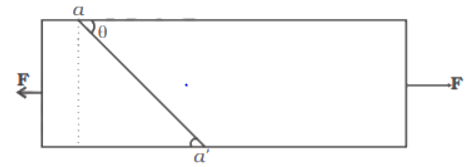# Mcq on mechanical properties of fluids class 11

Check out MCQ on mechanical properties of fluids for Class 11 Physics. This covers the topic like Stress, strain, Hooke’s law, Elastic energy etc. The Types of questions are True/False, Assertion and Reason, Multiple choice questions

I hope you will enjoy this test

General Instructions

1. Your test contains multiple-choice questions with only one answer type of questions. There are a total of 20 questions
2. This is a 45 min test. Please make sure you complete it in the stipulated time
3. You can finish this test any time using the 'Submit' button.

1. Which of the following has no dimensions?
2. Longitudinal strain is possible in
3. A stretched rubber has
4. A spring is stretched by applying a load to its free end.The strain produced in the spring is
5. The length of the metal wire  is a when the tension is T1 and b when the tension is T2. The original length of the wire is
6. A wire of length L and cross-sectional area A is made of a material of Young’s modulus Y, if the wire is stretched by an amount y, the work done is
7. The length of the two wires made of the same material having equal cross-sectional areas is L and 2L. They are stretched by the equal force F along the length. What will be the ratio of tension developed in the wires?
8. Two wires are made of the same material. The Length of the first wire is half that of the second wire and the radius of the first wire is twice that of the second wire. If the same  weight W is suspended  from the two wires, the ratio of the increase in their lengths is
9. The dimension of the Modulus of rigidity $\eta$ is
10. A solid sphere of radius R made of a material of bulk modulus K is surrounded by a liquid in a cylindrical container. A massless piston of area A floats on the surface of the liquid. When a mass M is placed on the piston to compress the liquid, the fractional change in the radius of the sphere is
11. (a) If both assertion and reason are true and reason is the correct explanation of the assertion.
(b) If both assertion and reason are true but reason is not correct explanation of the assertion.
(c) If assertion is true, but reason is false.
(d) If both assertion and reason are false.

Assertion: Lead is more elastic than rubber.

Reason: If the same load is attached to lead and rubber wires of the same cross-sectional area, the strain of lead is very much less than that of rubber
12. (a) If both assertion and reason are true and reason is the correct explanation of the assertion.
(b) If both assertion and reason are true but reason is not correct explanation of the assertion.
(c) If assertion is true, but reason is false.
(d) If both assertion and reason are false.
(e) If reason is true but assertion is false

Assertion: Stress is the internal force per unit area of abody.
Reason: Rubber is more elastic than steel.
13. (a) If both assertion and reason are true and reason is the correct explanation of the assertion.
(b) If both assertion and reason are true but reason is not correct explanation of the assertion.
(c) If assertion is true, but reason is false.
(d) If both assertion and reason are false.
(e) If reason is true but assertion is false

Assertion: Modulus of elasticity of most of the materials decrease with an increase in temperature

Reason: The interatomic forces of attraction becomes weaker as the temperature is increased
14. (a) If both assertion and reason are true and reason is the correct explanation of the assertion.
(b) If both assertion and reason are true but reason is not correct explanation of the assertion.
(c) If assertion is true, but reason is false.
(d) If both assertion and reason are false.
(e) If reason is true but assertion is false

Assertion: Young’s modulus for a perfectly plastic body is zero.
Reason: For a perfectly plastic body, restoring force is zero
15. A thin rod of negligible mass whose area of cross-section is $5\times 10^{-6} m^2$, is suspended vertically from its one end. Its length is 1 m at a temperature of $100^0C$. The rod is now cooled to $0^0C$but is prevented from contracting by suspended a mass at its lower end.

Young's modulus of the material of the rod is $2 \times 10^{11} N/m^2$ and coefficient of thermal expansion =$10^{-5} K^{-1}$ and g=10N/kg

Find the value of the suspended mass?
16. The energy stored in the rod will be
17. For a given material, the Young’s modulus is 2.4 times that of the rigidity modulus. The Poissons’s ratio is
18. For an ideal liquid, four statements are made
(i) the bulk modulus is infinite.
(ii) the bulk modulus is zero.
(iii) the shear modulus is infinite.
(iv) the shear modulus is zero

Which of the following are true

(a) (i) and (iii)

(b) (ii) and (iv)

(c) (ii) and (iii)

(d) (i) and (iv)
19. Consider a long steel bar under tensile stress due to forces F acting at the edges along the length of the bar  Consider a plane making an angle θ with the length. What is the tensile and shearing stresses on this plane?For what angle is the tensile stress a maximum?
20. For what angle is the shearing stress a maximum?

Subscribe
Notify ofThis site uses Akismet to reduce spam. Learn how your comment data is processed.